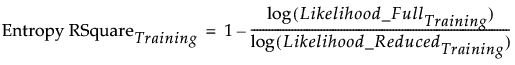Publication date: 07/30/2020

# Measures of Fit

## Entropy RSquare

The Entropy RSquare is computed for the training set and for the validation and test sets if validation is used. For the training set, Entropy RSquare is computed as follows:

The specified model is fit using the training set.

Predicted probabilities for each level of the response are obtained, based on the model.

Using these predicted probabilities, the likelihood is computed for observations in the training set. Call this Likelihood_FullTraining.

The reduced model (no predictors) is fit using the training set.

The predicted probabilities for the levels of the response from the reduced model are used to compute the likelihood for observations in the training set. Call this quantity Likelihood_ReducedTraining.

The Entropy RSquare for the training set is:The Entropy RSquare for the validation and test sets are computed in a manner analogous to the Entropy RSquare for the training set.